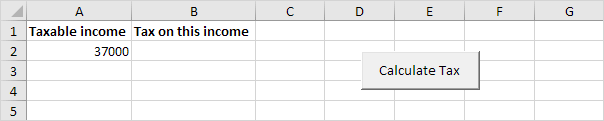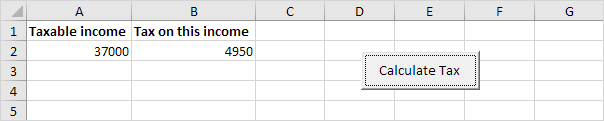# Tax Rates

Below we will look at a program in Excel VBA that calculates the tax on an income. The following tax rates apply to individuals who are residents of Australia.

Taxable income

Tax on this income

0 - \$6,000

Nil

\$6,001 - \$35,000

15c for each \$1 over \$6,000

\$35,001 - \$80,000

\$4,350 plus 30c for each \$1 over \$35,000

\$80,001 - \$180,000

\$17,850 plus 38c for each \$1 over \$80,000

\$180,001 and over

\$55,850 plus 45c for each \$1 over \$180,000

Situation:1. First, we declare two double variables. One double variable we call income, and one double variable we call tax.

Dim income As Double
Dim tax As Double

2. We initialize the variable income with the value of cell A2 and round it.

income = Round(Range("A2").Value)

3. We place the rounded value into cell A2 again.

Range("A2").Value = income

4. We use the Select Case statement to calculate the tax on an income. Excel VBA uses income to test each subsequent Case statement to see if the code under the Case statement should be executed.

Select Case income
Case Is >= 180001
tax = 55850 + 0.45 * (income - 180000)
Case Is >= 80001
tax = 17850 + 0.38 * (income - 80000)
Case Is >= 35001
tax = 4350 + 0.3 * (income - 35000)
Case Is >= 6001
tax = 0.15 * (income - 6000)
Case Else
tax = 0
End Select

Example: if income is 37000, tax equals 4350 + 0.3 * (37000-35000) = 4350 + 600 = \$4950

5. We write the value of the variable tax to cell B2.

Range("B2").Value = tax

6. Place this code in a command button and test it.

Result:Go to Next Chapter: Loop